# Cryptography Transposition Techniques

In this tutorial, we will learn about some transposition techniques which includes Rail-fence Technique, Columnar Transposition, Verman Cipher, and Book/Running-key Cipher. Also, we study their respective Algorithms. By Himanshu Bhatt Last updated : May 24, 2023

Transposition Techniques are based on the permutation of the plain-text instead of substitution.

## 1) Rail-Fence Technique

This technique is a type of Transposition technique and does is write the plain text as a sequence of diagonals and changing the order according to each row.

It uses a simple algorithm,

1. Writing down the plaintext message into a sequence of diagonals.
2. Row-wise writing the plain-text written from above step.

Example,

Let's say, we take an example of “INCLUDEHELP IS AWESOME”.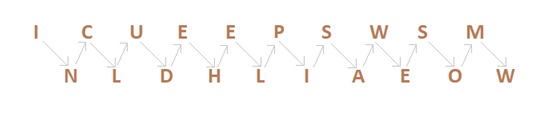So the Cipher-text are, ICUEEPSWSMNLDHLIAEOW.

First, we write the message in a zigzag manner then read it out direct row-wise to change it to cipher-text.

Now as we can see, Rail-Fence Technique is very to break by any cryptanalyst.

## 2) Columnar Transition Technique

A. Basic Technique

It is a slight variation to the Rail-fence technique, let's see its algorithm:

1. In a rectangle of pre-defined size, write the plain-text message row by row.
2. Read the plain message in random order in a column-wise fashion. It can be any order such as 2, 1, 3 etc.
3. Thus Cipher-text is obtained.

Let's see an example:

Original message: "INCLUDEHELP IS AWESOME".

Now we apply the above algorithm and create the rectangle of 4 columns (we decide to make a rectangle with four column it can be any number.)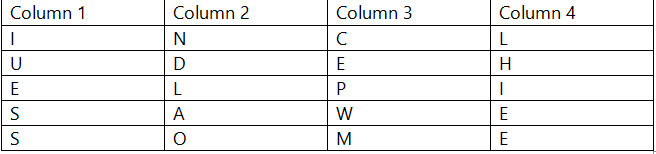Now let's decide on an order for the column as 4, 1, 3 and 2 and now we will read the text in column-wise.

Cipher-text: LHIEEIUESSCEPWMNDLAO

B. Columnar Technique with multiple rounds

In this method, we again change the chipper text we received from a Basic technique that is in round 1 and again follows the same procedure for the cipher-text from round 1.

Algorithm:

1. In a rectangle of pre-defined size, write the plain-text message row by row.
2. Read the plain message in random order in a column-wise fashion. It can be any order such as 2, 1, 3 etc.
3. Thus, Cipher-text of round 1 is obtained.
4. Repeat from step 1 to 3.

Example:

Original message: "INCLUDEHELP IS AWESOME".

Now we apply the above algorithm and create the rectangle of 4 column (we decide to make a rectangle with four column it can be any number.)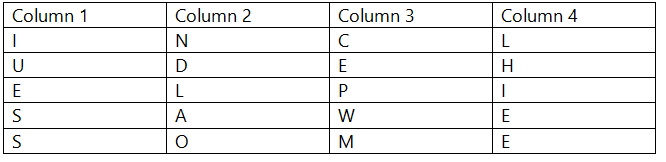Now let's decide on an order for the column as 4, 1, 3 and 2 and now we will read the text in column-wise.

Cipher-text of round 1: LHIEEIUESSCEPWMNDLAO

Round 2: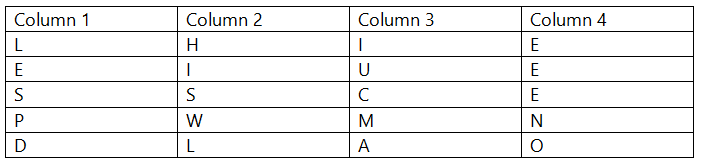Now, we decide to go with a previous order that is 4,1,3,2.

Cipher-text: EEENLESPICUMHISW

These multi-round columnar techniques are harder to crack as compared to methods seen earlier.

## 3) Vernam Cipher (One-Time Pad)

The Vernam Cipher has a specific subset one-time pad, which uses input ciphertext as a random set of non-repeating character. The thing to notice here is that, once an input cipher text gets used it will never be used again hence one-time pad and length of cipher-text is the size that of message text.

Algorithm:

1. Plain text character will be represented by the numbers as A=0, B=1, C=2,... Z=25.
2. Add each corresponding number of a plain text message to the input cipher text alphabet numbers.
3. If the sum is greater than or equal to 26, subtract 26 from it.
4. Translate each number back to corresponding letters and we got our cipher text.

Example:

Our message is "INCLUDEHELP" and input cipher text is "ATQXRZWOBYV"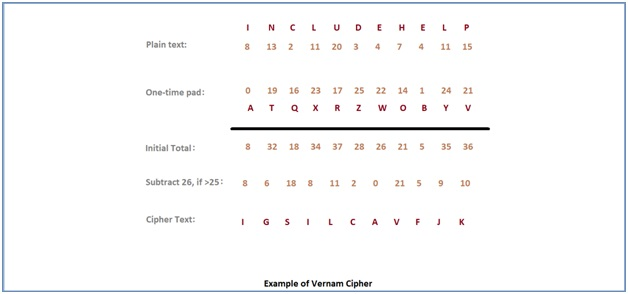One time pad should be discarded after every single use and this technique is proved highly secure and suitable for small messages but illogical if used for long messages.

## 4) Book/Running-Key Cipher

This technique also (incorrectly) known as running key cipher. This technique very simple and similar to our previous Vernam Cipher. For getting a cipher, some portion of text from a book is used as a one-time pad, rest it works in same way as Vernam cipher does.